## 3.4.3 Calling of module GEL3D1

### Description of the data

Different options are available to define the geometry, the splitting of the elements and the physical attributes.

• The geometry: option IOPTM, arrays X,Y and H, functions F1, F2 and F3:

• IOPTM = 0: the mesh is of finite difference type with constant step in the 3 directions. X, Y and H are three real values (declared as arrays) which represent the 3 spacing steps in the 3 directions. Every point, M, is referenced by its three indices I, J, and K and has coordinates: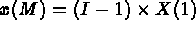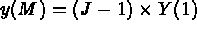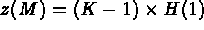• IOPTM = 1: the mesh is of finite difference type with variable step in the 2 first directions and constant step in the third direction. X an Y are two arrays of lengths N1 and N2, H is real (declared as an array); these values enable us to find the coordinates of each point, M, with indices I, J and K, as follows: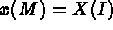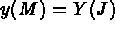• IOPTM = 2: the mesh is of finite difference type with variable step in the 3 directions. X, Y and H are three arrays of lengths N1, N2 and NB+1; these values enables us to compute the coordinates of any point M, with indices I, J and K, as follows: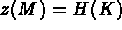• IOPTM = 3: the mesh is of finite difference type with variable step in the 2 first directions and a constant step in the third direction. X and Y are two arrays of length N1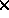N2 and H is real (declared as an array); these values are used to compute the coordinates of any point M, with indices I, J and K, as follows: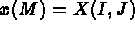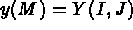• IOPTM = 4: the mesh is of finite difference type with variable step in the 2 first directions and a variable step in the third direction. X and Y are two arrays of length N1N2 and H is an array of length NB+1; these values are used to compute the coordinates of any point M, with indices I, J and K, as follows:• IOPTM = 5: a mesh of finite difference type with constant step in the 2 first directions used to construct the final mesh via the definition of points given by functions F1, F2 and F3. X and Y are real (declared as arrays). we obtain the following construction: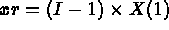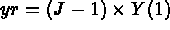and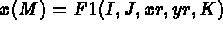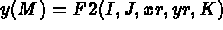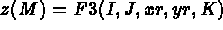• IOPTM = 6: a mesh of finite difference type with variable step in the 2 first directions used to construct the final mesh via the definitions of the points given by functions F1, F2 and F3. X and Y are two arrays of lengths N1 and N2. We obtain the following construction: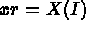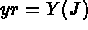and• IOPTM = 7: a mesh of finite difference type with variable step in the first 2 directions used to construct the final mesh via the definitions of the points given by functions F1, F2 and F3. X and Y are two arrays of lengths N1N2. We obtain the following construction: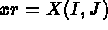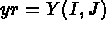and• IOPTM = < 0: a mesh of finite difference type with variable step in the 3 directions used to construct the final mesh via the definitions of the points given by arrays with indices X, Y and H (without verification).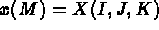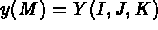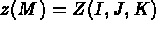• The splitting of elements: option NOPTDE:
• NOPTDE = 0: the result consists of hexahedra formed logically;
• NOPTDE = 1: each hexahedron is split into 2 pentahedra according to the direction of the first bisector in the z=0 plane;
• NOPTDE = 2: each hexahedron is split into 2 pentahedra according to the direction of the other diagonal;
• NOPTDE = 3: we first follow case NOPTDE = 1, then each pentahedron is split into 3 tetrahedra;
• NOPTDE = 4: the same with option NOPTDE = 2;
• NOPTDE = 5: each hexahedron is split into 5 tetrahedra.

• The physical attributes: sub-domain and reference numbers:
• the sub-domain numbers: option JOPTSD and arrays NUMAT and ITYP: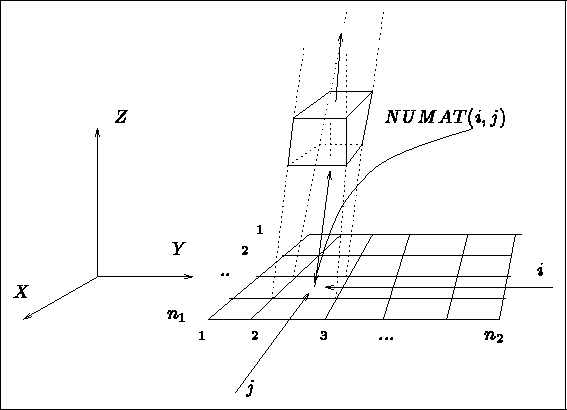Figure 3.28: Assignment of sub-domain numbers

• JOPTSD < 0: each element has the value -JOPTSD as sub-domain number.

• JOPTSD = 1: the domain is constituted of identical bands in terms of materials. Array NUMAT(1:N1,1:N2) associates the value NUMAT(I,J), as sub-domain number, to all the elements whose map on the z=0 plane is the element in position I, J.

• JOPTSD = 2: the domain (hexaheral elements) is constituted of different bands in terms of materials.

Array NUMAT(1:N1,1:N2,1:max(NTYP)) and array ITYP(1:NB) associates the value NUMAT(I,J,NTYP), as sub-domain number, to all the elements whose map on the z=0 plane is the element in position I, J, where NTYP = ITYP(K) (i.e. NTYP is the type of band K).

• JOPTSD = 3: the domain (pentahedral or tetrahedral elements) is constituted of identical bands in terms of the materials. Array
 NUMAT(2(1:N1),1:N2) associates the value NUMAT(I,J), as sub-domain number, to all the elements whose map on the z=0 plane is one of the 2 triangles in position I,J.

• JOPTSD = 4: the domain (pentahedral or tetrahedral elements) is constituted of different bands in terms of materials. Array
 NUMAT(2(1:N1),1:N2,1:max(NTYP)) and array ITYP(1:NB) associates the value NUMAT(I,J,NTYP), as sub-domain number, to all the elements whose map on the z=0 plane is one of the 2 triangles in position I, J, where NTYP = ITYP(K) (i.e. NTYP is the type of band K).

• The reference numbers (see figure 3.29):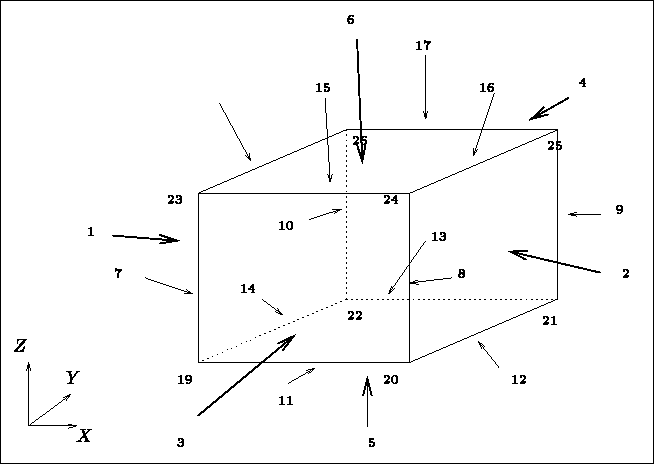Figure 3.29: Assignment of the reference numbers

• points: the points of the final mesh are, either the vertices of the topological cube, or points on its edges, or points on its faces, or lastly, internal points. Consequently, they have the following successive reference numbers: 19 to 26, 7 to 18, 1 to 6, or 0.
• edges: the edges of the final mesh are, either supported by the edges of the topological cube, or supported by its faces, or lastly, internal edges. Consequently, they have the following successive reference numbers: 7 to 18, 1 to 6, or 0.
• faces: the faces of the final mesh are, either supported by the faces of the topological cube, or are internal faces. Consequently, they have the following successive reference numbers: 1 to 6, or 0.

The point numbering is done according to the indications depicted in figure 3.30. The element numbering is done analogously, layer by layer.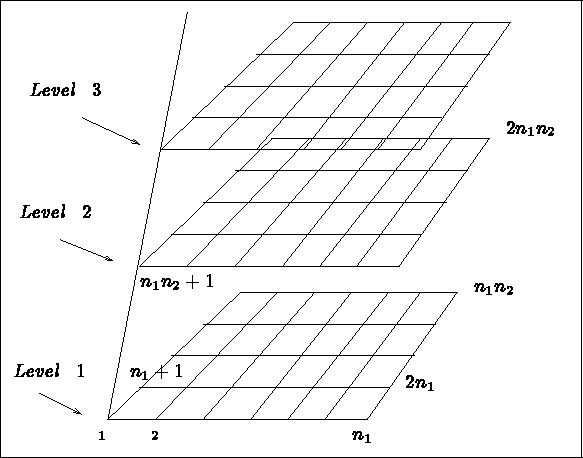Figure 3.30: Point numbering by module GEL3D1

### Calling the module

To call module GEL3D1 , it is necessary to write a main program as follows:

• dimension array M (in the blank common)
• declare the integer array NUMAT(.), array with 2 or 3 indices according to the case
• declare the integer array ITYP(NB) where NB is the number of layers
• declare the real array X(.), array with 1, 2 or 3 indices according to the case
• declare in the same way the real arrays Y(.) and H(.)
• declare the externals F1, F2 and F3 (according to the case)
• call INITI

• call the module

CALL GEL3D1 (M,IOPTM,JOPTSD,NOPTDE,N1,N2,NB,X,Y,H,
. F1,F2,F3,NUMAT,ITYP,NFNOPO,NINOPO,NFAUX)

where

• M is the super array
• IOPTM is the data description option
• JOPTSD is the sub-domain numbering option
• NOPTDE is the element splitting option
• N1 is the number of points in the x direction
• N2 is the number of points in the y direction
• NB is the number of layers (i.e. the number of points-1 in the z direction)
• X,Y and H are the arrays with 1, 2 or 3 indices to define the coordinates of the points
• F1, F2 and F3 are the names of the 3 external functions to supply (according to the value of IOPTM) to define the point coordinates. These functions have the following form:
• F1(I,J,XR,YR,K) defines the abscissis of the points from I and J, the position of the point in the plane of definition, XR and YR, the coordinates of point with indices I and J, and K , the sectional index (i.e. K=1,NB+1)
• F2(I,J,XR,YR,K) has the same form and defines the ordinates of the points
• F3(I,J,XR,YR,K) has the same form and defines the sides of the points
• NUMAT is an array with 2 or 3 indices to assign the sub-domain numbers
• ITYP is the array determining the type of layers (array linked to NUMAT)
• NF(NI)NOPO is the support number of the O.D.S. NOPO and its level
• NFAUX has the value 0

Remark:

In order to change the references which are automatically assigned, either module MODNOP, or preprocessor MODNXX, or the keyword NUME of APNOP3, can be used.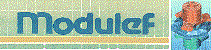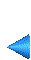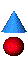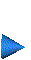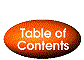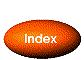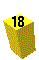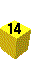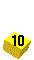Next: 3.4.4 Examples of use Up: 3.4 Method for a hexahedral topology Prev: 3.4.2 Description of the input data Index Contents